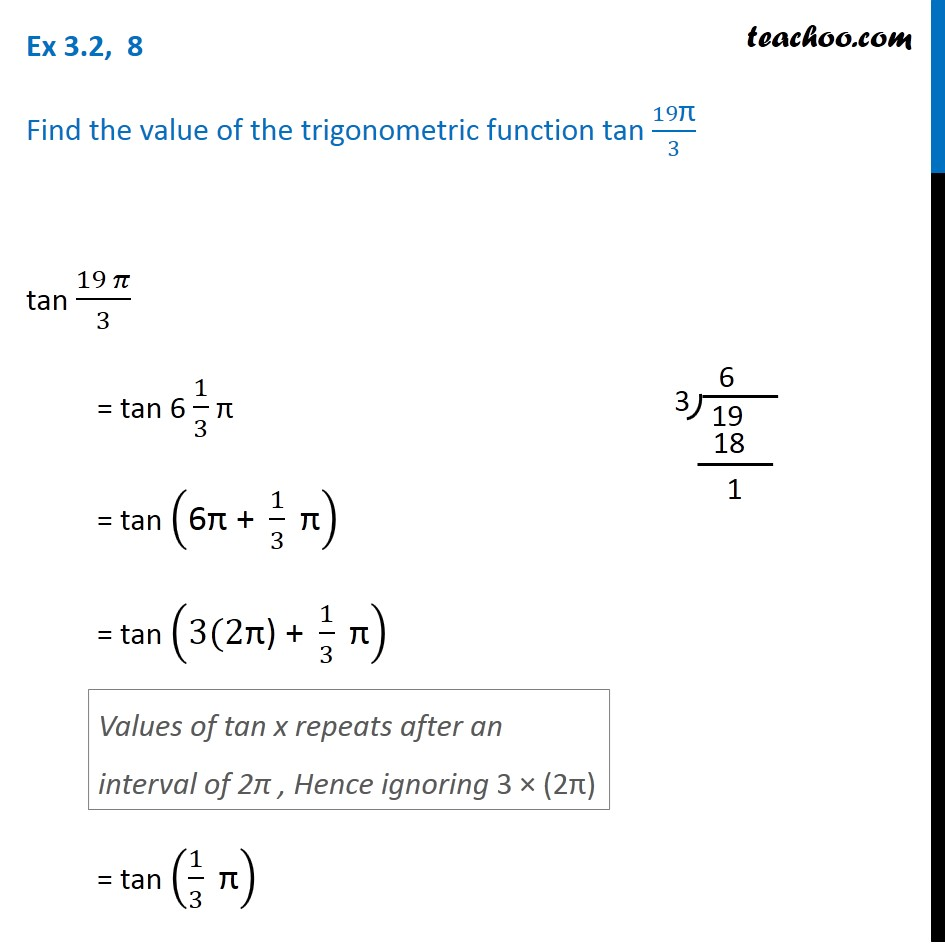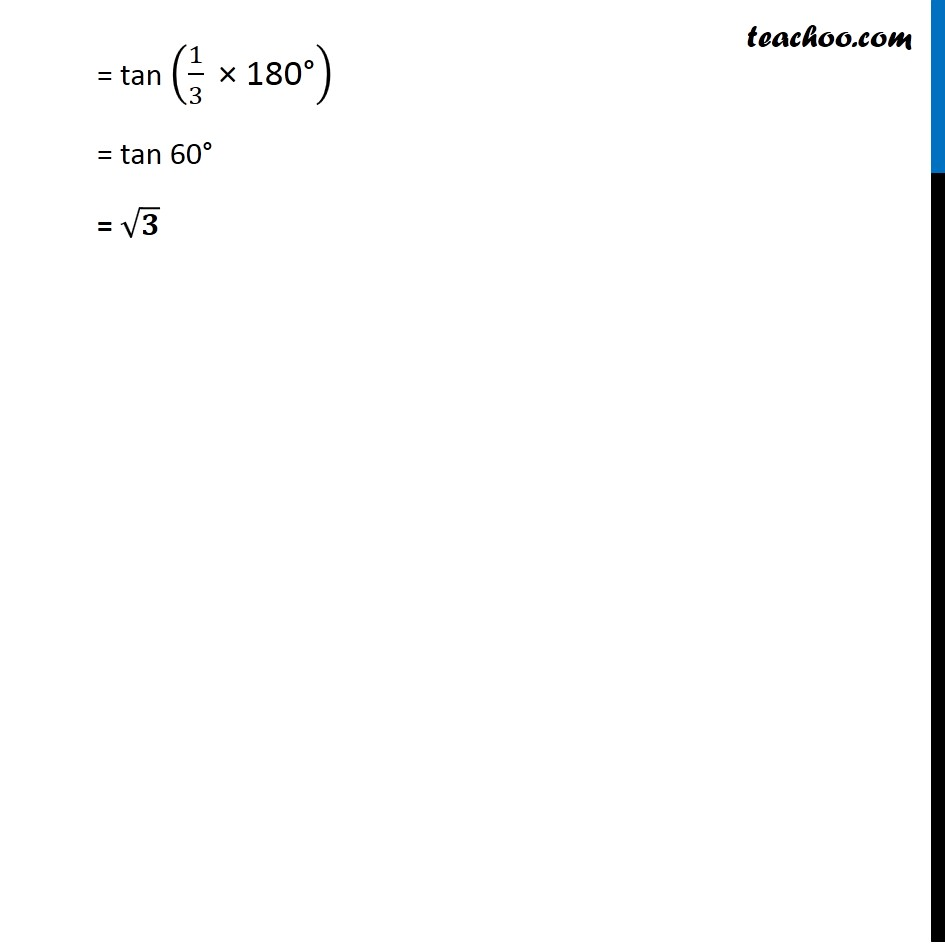Learn All Concepts of Chapter 2 Class 11 Relations and Function - FREE. Check - Trigonometry Class 11 - All Concepts1. Chapter 3 Class 11 Trigonometric Functions
2. Concept wise
3. Finding Value of trignometric functions, given angle

Transcript

Ex 3.2, 8 Find the value of the trigonometric function tan 19"π" /3 tan (19 𝜋)/3 = tan 6 1/3 π = tan ("6π + " 1/3 " π" ) = tan ("3(2π) + " 1/3 " π" ) = tan (1/3 " π" ) Values of tan x repeats after an interval of 2π , Hence ignoring 3 × (2π) = tan (1/3 " × 180°" ) = tan 60"°" = √𝟑

Finding Value of trignometric functions, given angle

About the AuthorDavneet Singh
Davneet Singh is a graduate from Indian Institute of Technology, Kanpur. He has been teaching from the past 9 years. He provides courses for Maths and Science at Teachoo.